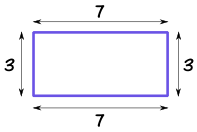Definition of

# PerimeterThe distance around a two-dimensional shape.

Example: the perimeter of this rectangle is 3+7+3+7 = 20

The perimeter of a circle is called the circumference.